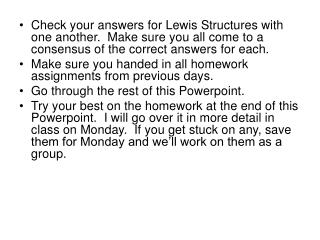# Last Topic of Chapter Bond Enthalpy - PowerPoint PPT PresentationDownload PresentationLast Topic of Chapter Bond Enthalpy

Last Topic of Chapter Bond Enthalpy
Download Presentation## Last Topic of Chapter Bond Enthalpy

- - - - - - - - - - - - - - - - - - - - - - - - - - - E N D - - - - - - - - - - - - - - - - - - - - - - - - - - -
##### Presentation Transcript

1. Check your answers for Lewis Structures with one another. Make sure you all come to a consensus of the correct answers for each. • Make sure you handed in all homework assignments from previous days. • Go through the rest of this Powerpoint. • Try your best on the homework at the end of this Powerpoint. I will go over it in more detail in class on Monday. If you get stuck on any, save them for Monday and we’ll work on them as a group.

2. Last Topic of Chapter Bond Enthalpy

3. Bond length Bond energy C C 154 pm 346 kJ/mol C C 134 pm 602 kJ/mol C C 120 pm 835 kJ/mol Since this bond is stronger, it requires more energy to break this bond. Bond Enthalpy Energy needed to break a bond in 1 mole of a gaseous substance.

4. The strength of a bond is also related to it’s stability. Consider N2 versus Cl2. N N Cl Cl Bond Length 1.10 A Bond Enthalpy 941 KJ / mol More Stable Bond Length 1.96 A Bond Enthalpy 242 KJ / mol Less Stable

5. *Bond enthalpies are determined experimentally using calorimetry. *Determining bond enthalpies for diatomic molecules is a straight forward experimental process. *When dealing with large molecules, individual bond enthalpies can be calculated by considering the average of each bond in the molecule Example:

6. ΔH of breaking methane into it’s original atoms (atomization) is experimentally determined to be 1660 KJ / mol H H C H C (g) + 4H (g) H Average bond enthalpy of C-H bond is therefore 1660 / 4 = 415 KJ/ mol Experiments of other C-H containing molecules yield similar results.

7. A Return to Hess’s Law *Bond enthalpies can be used to determine heats of reactions ΔHrxn. *Allows us to see what causes a reaction to be endo. or exo. Basic Concept Reactant + Reactant  Product + Product Energy required to Break Bonds Energy released when new bonds form The difference between the two determines endothermic versus exothermic.

8. Bond Energy • Table 8.4 lists values of some bond energies. • (Handout) • Should be over on heater by window. • To illustrate, let’s estimate the heat of reaction for reaction between methane and chlorine gas. CH4 (g) + Cl2 (g)  CH3Cl (g) + HCl (g)

9. CH4 (g) + Cl2 (g)  CH3Cl (g) + HCl (g) Calculation • In this reaction, one C-H bond and one Cl-Cl bond must be broken. • In turn, one C-Cl bond and one H-Cl bond are formed.

10. Notice: Only 1 C-H bond is broken on the left because ¾ C-H bonds appear as the same C-H bonds on the product side. Since these stay the same, there is no change in enthalpy associated with these.

11. Several Things to note: • Equation must be balanced. • Must draw Lewis Structures to see bonds between atoms. • 3) To calculate bond enthalpy, simply determine how many and of what type of bonds are broken on the left and how many and of what type of bonds are formed on the right. • Then calculate: Enthalpy of bonds broken – enthalpy of bonds formed. • 4) A single C-C bond has a different enthalpy than a double C=C bond. (true for all elements). • 5) Must look enthalpies up in tables. • 6) If a coefficient appears in the equation before a compound, you must multiply that coefficient by the bonds broken or formed.Ex: 2CH4 = 8 CH bonds broken. = 6(414) KJ

12. Homework 8.61-8.63, 8.65a, 8.66a

13. Bond Enthalpies Can Be Found in Data Tables